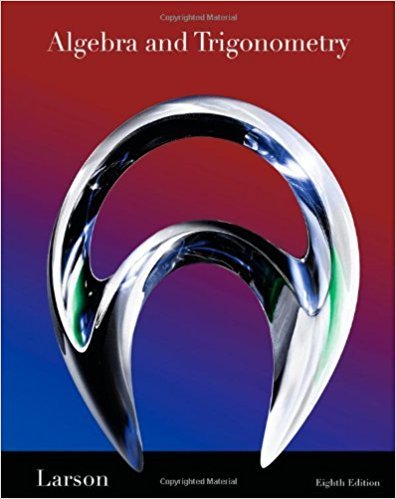×
×

# Solutions for Chapter 1.3: Modeling with Linear Equations## Full solutions for Algebra and Trigonometry | 8th Edition

ISBN: 9781439048474Solutions for Chapter 1.3: Modeling with Linear Equations

Solutions for Chapter 1.3
4 5 0 405 Reviews
12
0
##### ISBN: 9781439048474

Since 105 problems in chapter 1.3: Modeling with Linear Equations have been answered, more than 47506 students have viewed full step-by-step solutions from this chapter. Algebra and Trigonometry was written by and is associated to the ISBN: 9781439048474. This expansive textbook survival guide covers the following chapters and their solutions. This textbook survival guide was created for the textbook: Algebra and Trigonometry, edition: 8. Chapter 1.3: Modeling with Linear Equations includes 105 full step-by-step solutions.

Key Math Terms and definitions covered in this textbook
• Cholesky factorization

A = CTC = (L.J]))(L.J]))T for positive definite A.

• Complex conjugate

z = a - ib for any complex number z = a + ib. Then zz = Iz12.

• Determinant IAI = det(A).

Defined by det I = 1, sign reversal for row exchange, and linearity in each row. Then IAI = 0 when A is singular. Also IABI = IAIIBI and

• Diagonal matrix D.

dij = 0 if i #- j. Block-diagonal: zero outside square blocks Du.

• Elimination matrix = Elementary matrix Eij.

The identity matrix with an extra -eij in the i, j entry (i #- j). Then Eij A subtracts eij times row j of A from row i.

• Free variable Xi.

Column i has no pivot in elimination. We can give the n - r free variables any values, then Ax = b determines the r pivot variables (if solvable!).

• Incidence matrix of a directed graph.

The m by n edge-node incidence matrix has a row for each edge (node i to node j), with entries -1 and 1 in columns i and j .

• Iterative method.

A sequence of steps intended to approach the desired solution.

• Krylov subspace Kj(A, b).

The subspace spanned by b, Ab, ... , Aj-Ib. Numerical methods approximate A -I b by x j with residual b - Ax j in this subspace. A good basis for K j requires only multiplication by A at each step.

• Matrix multiplication AB.

The i, j entry of AB is (row i of A)·(column j of B) = L aikbkj. By columns: Column j of AB = A times column j of B. By rows: row i of A multiplies B. Columns times rows: AB = sum of (column k)(row k). All these equivalent definitions come from the rule that A B times x equals A times B x .

• Multiplication Ax

= Xl (column 1) + ... + xn(column n) = combination of columns.

• Norm

IIA II. The ".e 2 norm" of A is the maximum ratio II Ax II/l1x II = O"max· Then II Ax II < IIAllllxll and IIABII < IIAIIIIBII and IIA + BII < IIAII + IIBII. Frobenius norm IIAII} = L La~. The.e 1 and.e oo norms are largest column and row sums of laij I.

• Particular solution x p.

Any solution to Ax = b; often x p has free variables = o.

• Pascal matrix

Ps = pascal(n) = the symmetric matrix with binomial entries (i1~;2). Ps = PL Pu all contain Pascal's triangle with det = 1 (see Pascal in the index).

• Random matrix rand(n) or randn(n).

MATLAB creates a matrix with random entries, uniformly distributed on [0 1] for rand and standard normal distribution for randn.

• Rank one matrix A = uvT f=. O.

Column and row spaces = lines cu and cv.

• Reflection matrix (Householder) Q = I -2uuT.

Unit vector u is reflected to Qu = -u. All x intheplanemirroruTx = o have Qx = x. Notice QT = Q-1 = Q.

• Row picture of Ax = b.

Each equation gives a plane in Rn; the planes intersect at x.

• Skew-symmetric matrix K.

The transpose is -K, since Kij = -Kji. Eigenvalues are pure imaginary, eigenvectors are orthogonal, eKt is an orthogonal matrix.

• Stiffness matrix

If x gives the movements of the nodes, K x gives the internal forces. K = ATe A where C has spring constants from Hooke's Law and Ax = stretching.

×